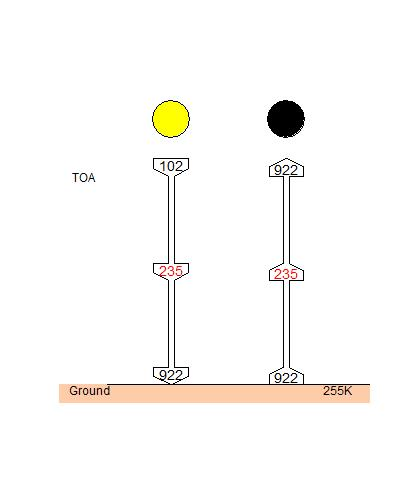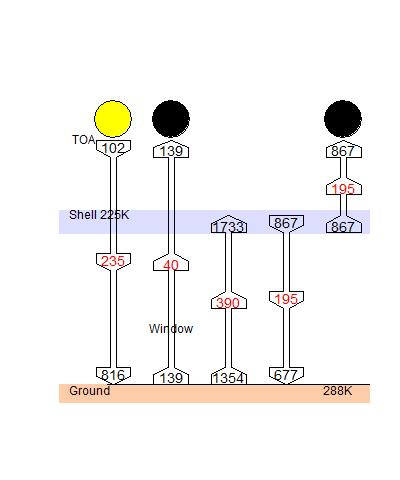## Saturday, October 2, 2010

### ## An entropy budget for the Earth

This post follows up some ideas in comments to the Science of Doom post on 2LoT. Whenever you calculate a heat flux, and you know the temperature, you can calculate the entropy changes. Tracking entropy is different from tracking energy flux, in that entropy is not conserved, but will be created whenever there is irreversible heat transfer. However, it is conserved in reversible processes, and can never decrease globally, although it can be transferred to somewhere else. And as with heat, for Earth steady state requires that entropy that enters or is created, must leave.

So I have the idea of doing an entropy budget, analogous to Trenberth's famous energy budget for the Earth. It's a bit more complicated though, so this post will look at a simplified Earth.

#### Entropy calculations

Whenever heat Q Joules is added to a region at constant temperature T, the entropy increases by Q/T J/K. If Q is negative (heat is lost), then so is entropy (to that region). If T is not constant, you have to integrate, but the idea is the same.

By the Second Law, if the heat flows from one region to another in an otherwise isolated system, the second must be the cooler, and it will gain more entropy than the first lost.

In these examples, we'll be dealing with fluxes, so Q is replaced by F, the flux in J/s/m^2, or W/m^2, and the result, S, is an entropy flux in W/K/m^2.

#### Entropy significance

The entropy associated with a heat flux is associated with its capacity to do work. In fact, a Helmholtz (or Gibbs - same since we're not considering PV work here) free energy can be defined:
A = F - TS
remembering that A, F and S are flux densities. A is a measure of the power that could be delivered as mechanical work from the heat flux F if discharged to a heat sink at temperature T.

This can be used as a more rigorous definition of the entropy flux associated with an E/M flux:
S=(F-A)/T,
where again, A is the mechanical power that could theoretically be generated by using the flux F with heat sink at T.

As a heat flux passes to cooler regions, it loses some capacity to do further work. However, in that transfer it could have done work if a heat engine mechanism existed (equivalent to the capacity it had subsequently  lost). So when you see entropy increasing, it means that where that happened, there was a capacity to generate weather effects, etc.

#### Snowball Earth

 I'll introduce a symbol for a heat/entropy flux. It's an arrow with three numbers. In the middle, in red, is the heat flux. At the tail, in black, is the entropy flux associated with the origin of the flux, and at the head is the entropy transferred to the heat sink. So here you see the basic calc for average fluxes for snowball Earth, with a clear atmosphere with no GHG. Incoming solar is 342 W/m2, but 107 W/m2 is reflected (assuming Earth's true albedo still applies), so at balance 235 W/m2 is emitted directly to space as IR. The surface temperature required to do that is 255K. Entropy (820 W/K/m2) is created when SW is thermalised at the surface. This is an irreversible process. The exchange could have driven a heat engine, but didn't. No further irreversible process occurs, and the entropy is exported to space unchanged.In this calculation the incoming temperature was assigned a value of 2310K. The apparent emission temperature from the sun is 5700K, so why the reduction?

It is a subtle point, but while incoming SW has the theoretical ability to heat a black body to 5700K (with concentrating mirrors etc), at that temperature all the energy is radiated back to space, and cannot be used to perform work. There is a discussion here. The optimum temperature for conversion of solar energy to work is 2310K.

The effect of the difference is slight - on either count, incoming entropy is small.

#### Earth with GHG shell

 GHG's in the atmosphere absorb and emit radiation, some of which returns to the surface, and some of which exits to space. Most of the 235 W/m2 received by the Earth's surface is returned to space emitted from GHG's at high altitude. The resulting transfers create entropy in a rather distributed way. However much of the effect is captured by a simplified model in which GHG's are confined to a narrow band near TOA, at 225K. This band has an atmospheric window, 40 W/m2. As with the Snowball Earth, that flux gains no further entropy. However, the proportion absorbed at the shell does yield an entropy change. Some returns to the warmer surface, with an entropy decrease. And some is emitted to space, with entropy loss to the Earth (and gain by space). The total export of entropy is 1006 W/K/m2.The entropy decrease has been the subject of argument at SoD. However, from a budget point of view, the up arrow from ground to shell, and the down arrow, could be merged, with a tail of 667 W/K/m2 and a head of 867, and a net entropy gain of 220 W/K/m2. This is what counts for 2LoT - the nett change in entropy, which is positive.

#### The Greenhouse Effect and entropy

Comparing with and without GHG:
• With GHG there is less entropy created by incoming SW at the warmer surface - 714 W/K/m2 vs 820.
• With GHG the total export of entropy is higher - 1006 W/K/m2 vs 922.
• The entropy gain from ground to shell of 220 W/K/m2 can be associated with heat engine effects in the atmosphere, or with life processes etc. The colder shell is necessary to export the difference.

#### Realistic?

Yes, in terms of budgetting. The shell artifice misrepresents the apparent temperature of the downwelling IR (at 225K) - it's much higher. The 190 W/K/m2 entropy reduction does not occur at the surface, but distributed through the atmosphere. Likewise, the upwelling stream entropy increase is likewise distributed, But this does not affect the budget.

And yes, I've ignored clouds.

#### 1 comment:

1.Nicely put, it makes me ponder the energy budget in these terms beneath an ice shelf.